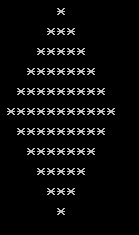Home >  > 人脑与电脑

## 人脑与电脑

0

```import math
for i in range(10000):
#转化为整型值
x = int(math.sqrt(i + 100))
y = int(math.sqrt(i + 268))
if(x * x == i + 100) and (y * y == i + 268):
print i
```

```l = []
for i in range(3):
x = int(raw_input('integer:\n'))
l.append(x)
l.sort()
print l
```

https://github.com/python/cpython/blob/master/Objects/listobject.c

```a = [1,2,3]
r = []
for each in a:
r.append(each+1)
```

```map(lambda x:x+1,[1,2,3])
```

```list(map(lambda x:x+1,[1,2,3]))
```

Map函数就是一种整体的思维，将[1,2,3]看作一个整体，而不再是单个的元素，这样就可以大大提高我们的效率，考虑事情的思维层级也会高出一些来。```[1, 2, 3, 5, 8, 13, 21, 34, 55, 89, 144, 233, 377, 610, 987, 1597, 2584, 4181, 6
765, 10946]
[2, 3, 5, 8, 13, 21, 34, 55, 89, 144, 233, 377, 610, 987, 1597, 2584, 4181, 6765
, 10946, 17711]
32.69
```

```a = 2.0
b = 1.0
s = 0
for n in range(1,21):
s += a / b
t = a
a = a + b
b = t
print(t,a,b)
print (s)
```

······ 6x-1，6x，6x+1，6x+2，6x+3，6x+4，6x+5，6(x+1），6(x+1)+1 ······